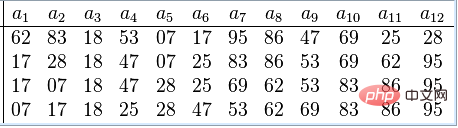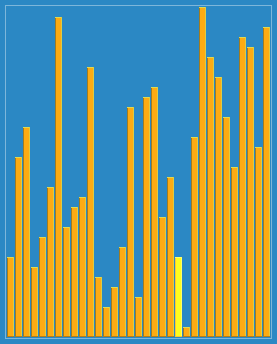# PHP希尔(Shell)排序算法的实现（代码详解）Shell排序示例如下：PHP实现希尔(Shell)排序算法的代码示例：

```<?php
function shell_Sort(\$my_array)
{
\$x = round(count(\$my_array)/2);
while(\$x > 0)
{
for(\$i = \$x; \$i < count(\$my_array);\$i++){
\$temp = \$my_array[\$i];
\$j = \$i;
while(\$j >= \$x && \$my_array[\$j-\$x] > \$temp)
{
\$my_array[\$j] = \$my_array[\$j - \$x];
\$j -= \$x;
}
\$my_array[\$j] = \$temp;
}
\$x = round(\$x/2.2);
}
return \$my_array;
}

\$test_array = array(3, 0, 2, 5, -1, 4, 1);
echo "原始数组 :\n";
echo implode(', ',\$test_array );
echo "\n排序后数组\n:";
echo implode(', ',shell_Sort(\$test_array)). PHP_EOL;```

```原始数组 : 3, 0, 2, 5, -1, 4, 1